Materialized Views (MV) in DBMS

Suppose we have one query which has few large tables and small tables joined, and this query is used most frequently by the user. What happens to the query and its performance each time the user fires it? The optimizer will evaluate it each time, using all above methods and tries to see the optimal cost and path to evaluate it. It traverses those big tables each time, causing the performance of the query down. But imagine, what will happen to the performance, if we have the result of those large table selections in some other table or view? The number of records will be reduced; it will reduce the cost of accessing large tables.

Above concept of reducing the cost and enhancing the performance of the query lead to the generation of materialized views. These views are created by using the queries and result of which are stored in the views. They act like any other tables. These views will be created and data will be stored much before the query is executed. The query will access this view like any other table and get the required records from it. It can participate in other queries too. It can be joined with any other tables, views or any other materialized views.

But these materialized views are not created at run time. They are compiled and executed beforehand itself. Hence if there are any new records added to the tables that query of materialized view uses, then the MV would not have updated with new records. It will have records before to new insertion. Hence keeping MV up-to-date is more important. Otherwise we will lose some records in the result. Keeping MV records up-to-date is called materialized view maintenance. We have to refresh the MV every time a new record is inserted / updated / deleted from underlying tables. But this would be a costly effort to refresh every time, because it is same as running underlying query again and again.

Instead of this, if we do the refresh to the MV in incremental order, it will reduce the time of refresh. We can perform incremental refresh in any of the following method

• Whenever there is an insert / delete / update on the underlying table, a trigger is written to fire and update the records in MV. i.e.; it will insert / delete / update the records that have been affected the tables in MV.
• Some procedure can be written to update MV whenever there is a DML on the tables.
• Periodic refreshing the database rather than refreshing it every time there is a change to the records in the table. i.e.; nightly once refresh or daily two times refreshing MV, so that it is up-to-date with underlying tables. This method may have little difference in records as MV is refreshed nightly or some other time. The records entered between two refresh will not be available, if there is any request during that time.

Now let us see how to incrementally refresh MVs when there is a change in underlying tables. The refreshing technique changes according to the operations used in MV query. Suppose T and S is the two tables involved in creating MV. Let table T has some changes – may be insert or delete and let Told and Tnew refer to the table before and after the changes to the table. Let Ri and Rd be the inserted set of records and deleted set of records. Now let us see different cases of incremental refresh of MVs below :

Join

Suppose we have MV as a result of joining table T and S. Suppose we have set of records inserted into table T. Now the incremental refresh will check for the newly inserted records and join only them with the table S and insert them to the MV. This is because; MV has already the old records as result of joining T and S. It needs only the newer inserts into it.

Let MV = T ∞S = MVold
Now MVnew = Tnew∞ S = (Told U Ri) ∞ S = (Told ∞ S) U (Ri∞ S) = MVold U (Ri∞ S)

Database Management System

All the transformation for getting this is based on the equivalence rule. Hence refreshed materialized view is the join between the newly inserted records and table S. This will have less cost as new set of records will be comparatively less.
Let us consider this based one example. Suppose we have a materialized view with student id, name, address, class id and class name from a query on STUDENT and CLASS table as shown below: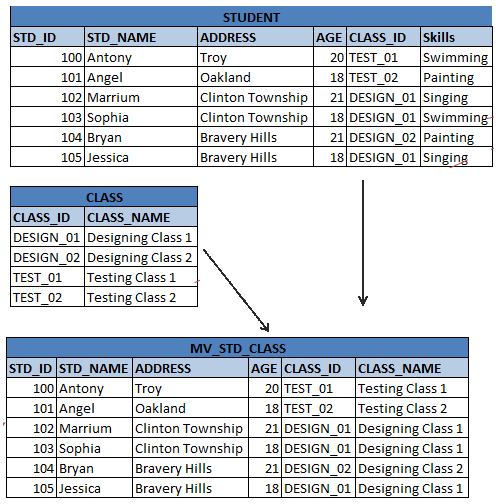Now let below rows are added to STUDENT table.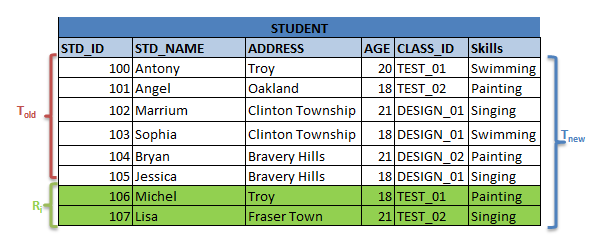It is clear from above diagram that we have to add only two new records to MV_STD_CLASS. Hence it can be done as below :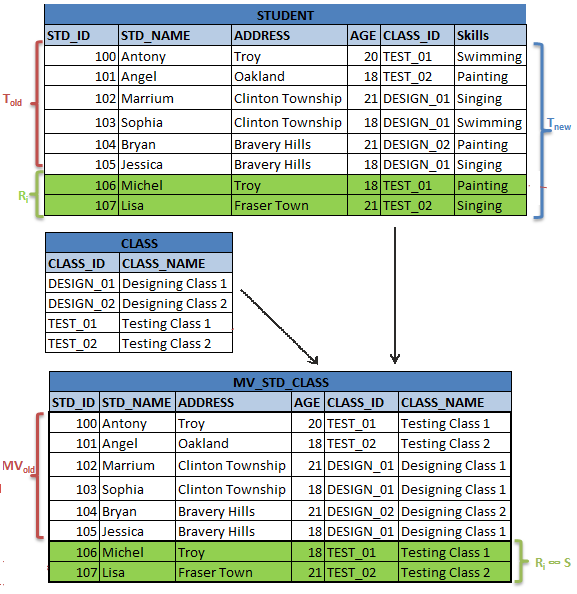Suppose some records are deleted from the table T. then the same procedure is repeated, but the deleted record is removed from the existing MV as below :

Let MV = T ∞S = MVold
Now MVnew = Tnew∞ S = (Told – Rd) ∞ S = (Told ∞ S) -(Rd∞ S) = MVold – (Rd∞ S)

Above example, while deleting would be as shown below :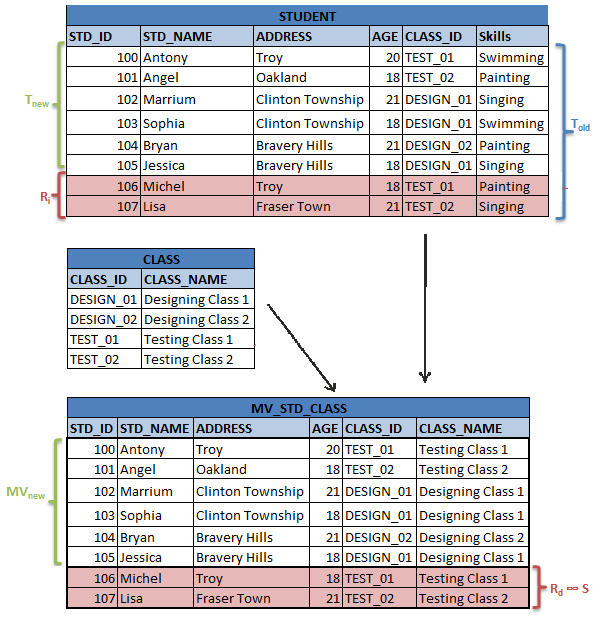Outer Joins

This is similar to join operations above. The only difference is that; it should look for extra records that do not exist in other tables. Hence initially it will perform the normal joins as above. Then it will take the records of first table that did not participate in the join and add it to MV with columns of other table as NULL.

Suppose we have MV = T ∞ left outer join S

When there are new records in table T, it will check in table S for matching records. If it exists, then it will add the records from both the tables. If no matches found, then it will add the records of first table to MV and make the other table columns NULL.

When records are deleted from table T, then it will remove the record from MV. If records are removed from S, then it will make the columns of S in MV as NULL instead of deleting the record from MV.

Selection and Projection

Suppose we have selection operation on table T, i.e.; we are filtering some set of records to get subset of T.   Suppose we have created MV on T for this case and we have some new records inserted into T. Then MV =σ θ (T) = MVold
Now new MV is : MVnew = σ θ (Tnew) = σ θ (Told U R i) = σ θ (Told) U σ θ (R i) = MVold U σ θ (R i)
i.e.; we have to add the new records to MV after applying selection on new records. Similarly, when we have delete operation, we have to delete the records after applying the selection operation on deleted records.
Now new MV after delete is : MVnew = σ θ (Tnew) = σ θ (Told -R d) = σ θ (Told) – σ θ(R d) = MVold – σ θ (Rd)

File Processing System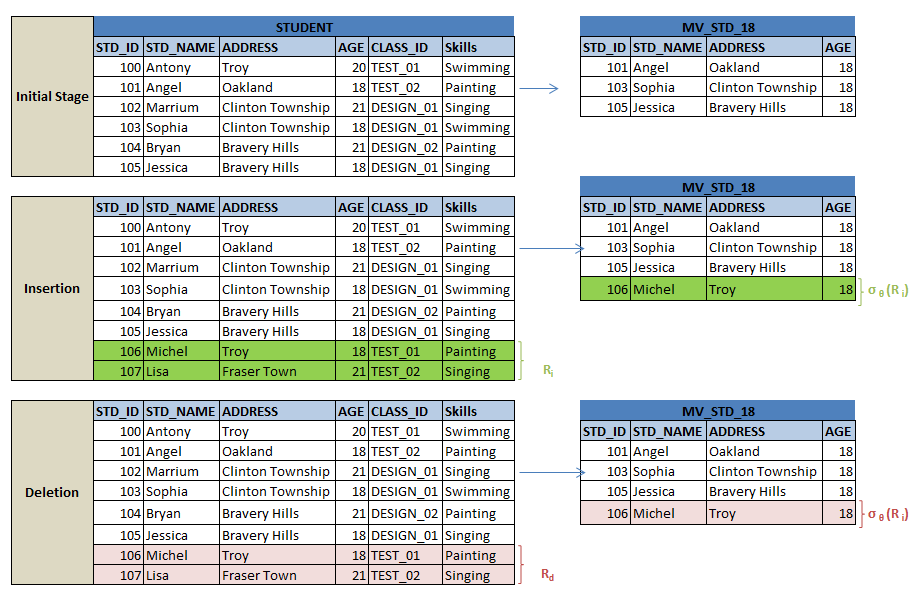Let us consider the projection operation. While applying projection operation, we select the columns from the table. Refreshing the MV with projection is different from above all cases. Here we don’t delete the records all the time, as it involves selection of columns. Here we simply keep the count of the columns, each time it is inserted or deleted. If it is entirely deleted from the table, then we delete the record from MV.

Whenever there is insertion of records into table, we check if the column is already present in MV and if yes, increment the count; else we add the new record and initialize the count to one.
When there is deletion of records, we decrement the count; when the count reaches zero, we delete the entire record from MV.

Let us understand using an example. Suppose we have a materialized view with distinct CLASS_ID from STUDENT table. Here we can have multiple records with same CLASS_ID in the table, and number of count of it will be kept for refreshing MV. See the below table diagram.
i.e.; MV = ∏CLASS_ID (STUDENT)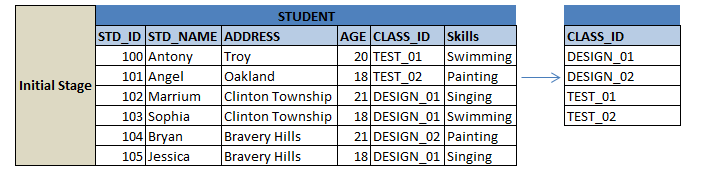The incremental refresh will keep the count for DESIGN_01 as 3, DESIGN_02 as 1, TEST_01 as 1 and TEST_02 as 1 in above case. They are the number of occurrences of them in the table STUDENT. Suppose we have new entry into table STUDENT. Then the MV would be :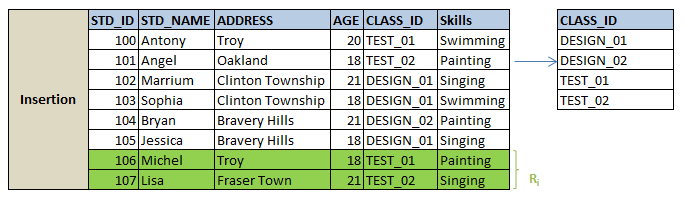We see that there is no difference to MV, as the new records have same column values as before. But the count of TEST_01 and TEST_02 is incremented to 2 to keep track of it.
Suppose we had new record with new CLASS_ID has been entered. Then the count of new CLASS_ID would be zero in its tracker, hence it adds it to MV and increments the count for it as one.

Architecture of Database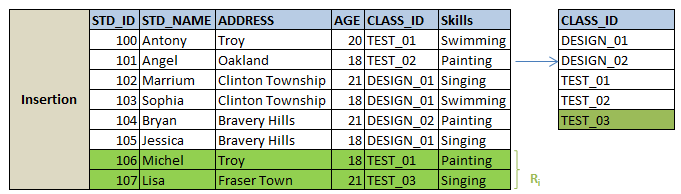Suppose we have selected STD_NAME and CLASS_ID in MV. Then in both cases above, the new record will be inserted into MV along with count increment.

Now let us start deleting the records from STUDENT, say we deleted student record with STD_ID= 100. Then MV would be :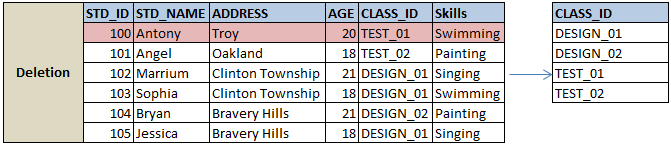We observe that there is no change to MV, but the count of DESIGN_01 is decremented to 2. Suppose we deleted a record DESIGN_02. Now it will decrement its count to zero. Since it is zero now, the record will be deleted from MV.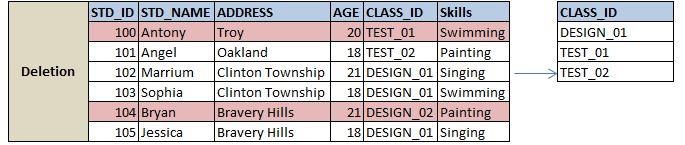Suppose we have selected STD_NAME and CLASS_ID in MV. Then in both cases of deletion above, respective records will be deleted from MV along with count decrement.

This is how the projection on MV gets refreshed.

Aggregation / Grouping

Here we have different types of aggregations like count, sum, max, min etc.

• Count : When we are calculating the number of records of each group, it should simply keep track of records as we did in projection, but it has to be added as column in MV. Suppose we inserted a new record into the table T. Then it will check if that new record already exists in MV. If exists, it increments its count by one, else it adds a new record to MV with count as 1. When there is deletion of records from table T, it decrements the count from MV, if exists. If the count reaches zero, it deletes that record from MV.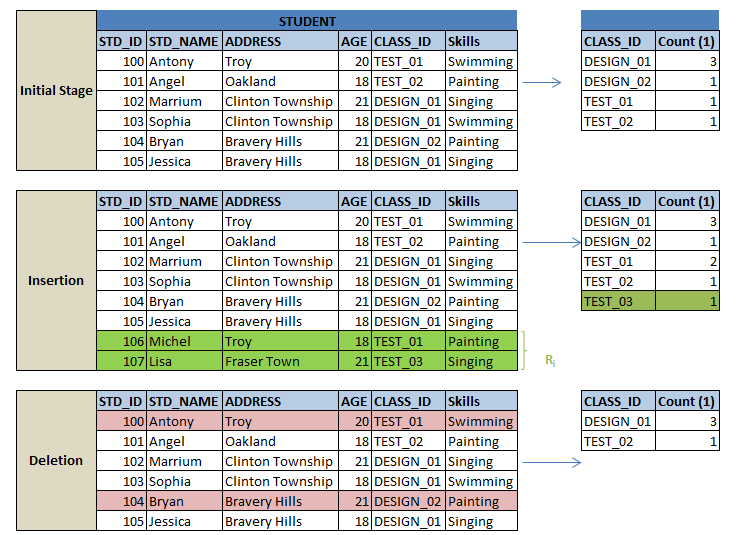• SUM : This aggregation is similar to count, but it adds/subtracts the value of columns instead of incrementing. In addition, it keeps the count of each group to track if it is fully deleted from the table and to delete it from MV, like in COUNT.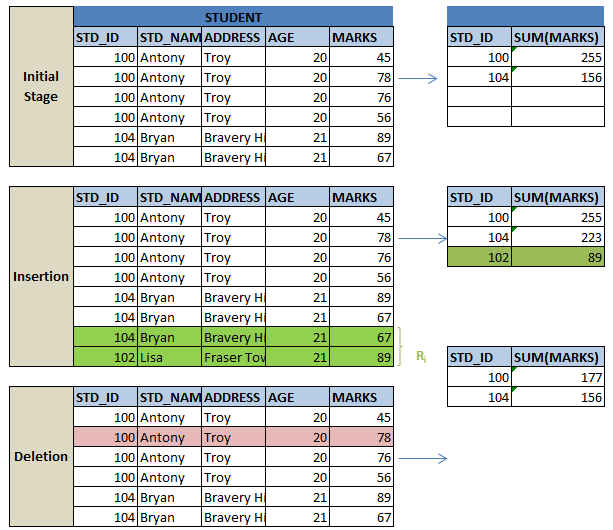• AVG : Here we perform both COUNT and SUM separately to calculate AVG.
• MAX and MIN : While finding the MAX or MIN, it will compare newly inserted value with the max or min value in MV and decide whether it has to update the value in MV accordingly. When we delete the record, it will compare with the value in MV and if deleted record is not max or min value, no effort is involved. But if the deleted record is max or min value, then it has to calculate MAX and MIN again from the table because it does not have the information of other records in table. This will be bit expensive work as it is similar to refreshing entire MV again.
Characteristics of a Database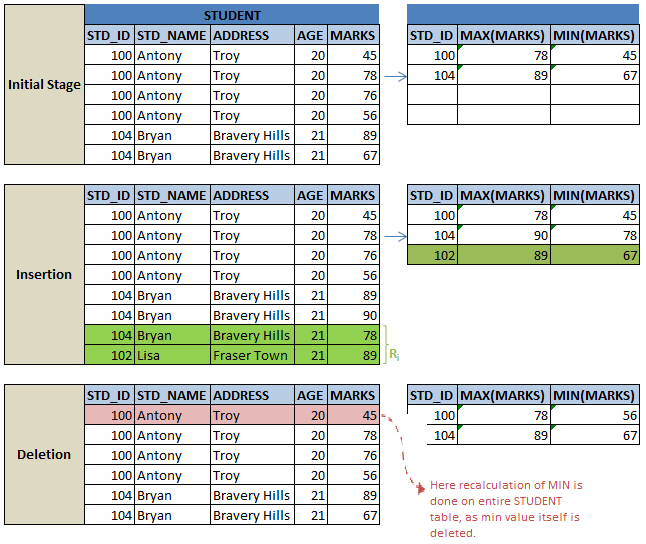Set INTERSECT, UNION and DIFFERENCE

All these set operations are handled in same manner.
Suppose MV is created by intersection of table T and S. When there is insertion of records into table T, then the new record is checked against table S to find out if it exists there. If yes, then it will be added to MV; else it will be ignored. When a record is deleted, it will be checked against S to find it exists in S too. If yes, then it will be removed from MV; else it will ignore it.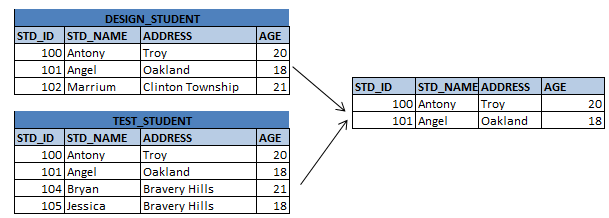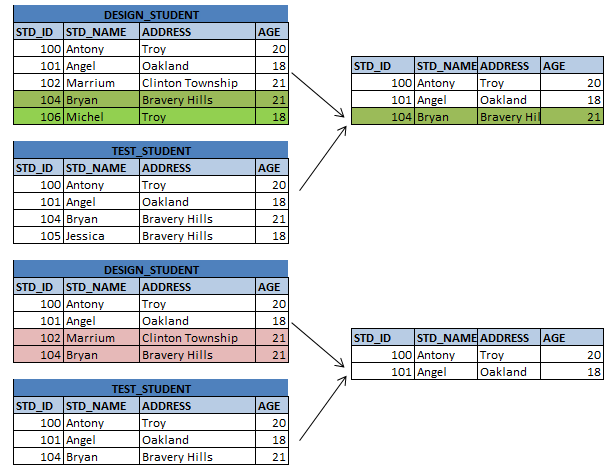Same is done when set operator union is used to create MV. When a new record is inserted, it checks MV for its existence. If it exists, then it ignores it; else it inserts the record to MV. When a record is deleted, it removes the record from MV if it exists; else ignores it.

Same is the case with set difference.

Expressions :

In expressions also, we can have incremental refresh of records like we saw above cases.
Suppose we have E1 ∞E2 where E1 and E2 and two expressions. Suppose R1 be the set of records added to E1. Updating the new records to the join between two expressions works same as joins between the tables. i.e.; rather than involving whole expressions again in joining, only the new set of records alone are involved in the join to get it updated. This will make the update faster.
New E1 ∞E2 = Old (E1 ∞E2) U (R1 ∞E2)

Materialized Views in Queries

Let us see how materialized views are helpful while evaluating the queries.
Suppose we have joins among three tables R, S and T. Suppose we have MV on two table T and S. Then the query can be modified to use MV as below :

Data Independence

MV = S ∞ T

Query = R ∞S ∞ T  → R ∞ MV

But using materialized view in all the cases would not be efficient. Suppose we do not have any index defined on MV. But we have index defined on the individual tables S and T of MV. Then in above case, rather than replacing the joins between S and T with MV, it would be better to use them as it is. Because it will use index to evaluate the joins and will be faster than MV.

Suppose we have tables STUDENT and CLASS upon which we have MV created. Suppose we have index on AGE of STUDENT table and CLASS_NAME of CLASS table. We have fired a query to get the records from STUDENT, CLASS and TEACHER tables. Let us represent the query in relational algebra as below:

Query : σ AGE= 21 AND CLASS_NAME = ‘DESIGN_01’ (STUDENT ∞ CLASS ∞ TEACHER)
MV : STD_CLS = STUDENT ∞ CLASS

Optimizer can have queries as below :

• σ AGE= 21 AND CLASS_NAME = ‘DESIGN_01’ (STD_CLS ∞ TEACHER)
• σ AGE= 21 (STUDENT)  ∞ σ CLASS_NAME = ‘DESIGN_01’ (CLASS) ∞ TEACHER

The first query uses MV to evaluate the expression. Second query uses the indexes of respective tables to evaluate them.  Compared to both the cases, second query will run faster as it uses the index. Although MV has reduced the cost of join between STUDENT and CLASS, it acts like non indexed table here. We need to select AGE and CLASS_NAME from MV, and it will traverse each record one by one for this condition. Whereas first query will directly fetch the records from respective table, and make its subset smaller and then it will perform the join. Hence first query is efficient.

Though we can replace the queries with MVs, it is the optimizer who calculates the statistics of each tables and MVs usage and decides which one to use for better performance.